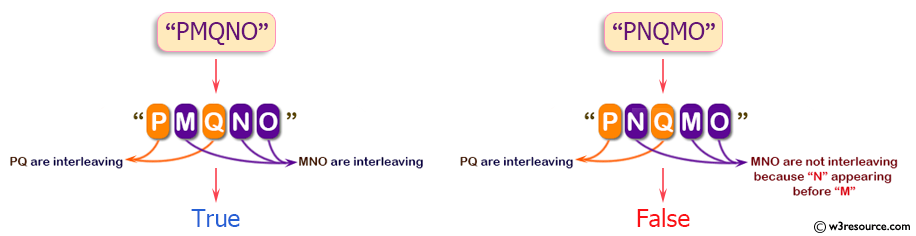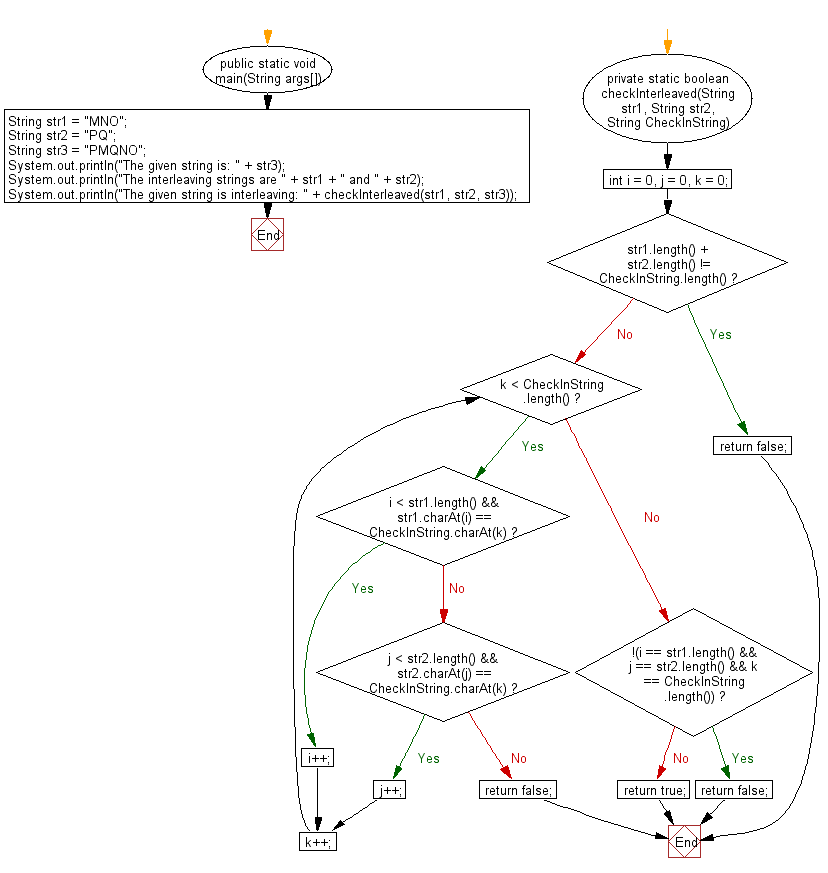﻿ Java exercises: Check whether two strings are interleaving of a specified string - w3resource# Java String Exercises: Check whether two strings are interleaving of a specified string

## Java String: Exercise-36 with Solution

Write a Java program to check whether two strings are interleaving of a given string. Assuming that the unique characters in both strings.

Pictorial Presentation:Sample Solution:

Java Code:

``````import java.util.*;
public class Main {
public static void main(String args[]) {
String str1 = "MNO";
String str2 = "PQ";
String str3 = "PMQNO";
System.out.println("The given string is: " + str3);
System.out.println("The interleaving strings are " + str1 + " and " + str2);
System.out.println("The given string is interleaving: " + checkInterleaved(str1, str2, str3));
}
private static boolean checkInterleaved(String str1, String str2, String CheckInString) {
int i = 0, j = 0, k = 0;
if (str1.length() + str2.length() != CheckInString.length()) {
return false;
}
while (k < CheckInString.length()) {
if (i < str1.length() && str1.charAt(i) == CheckInString.charAt(k)) {
i++;
} else if (j < str2.length() && str2.charAt(j) == CheckInString.charAt(k)) {
j++;
} else {
return false;
}
k++;
}
if (!(i == str1.length() && j == str2.length() && k == CheckInString.length())) {
return false;
}
return true;
}
}
```
```

Sample Output:

```The given string is: PMQNO
The interleaving strings are MNO and PQ
The given string is interleaving: true

The given string is: PNQMO
The interleaving strings are MNO and PQ
The given string is interleaving: false
```

N.B.: In the 2nd case, in interleave string, N comes before M and in original string str1 = MNO (N comes after M) So it is not interleaved.

Flowchart:Visualize Java code execution (Python Tutor):

Java Code Editor:

Improve this sample solution and post your code through Disqus

What is the difficulty level of this exercise?

﻿

## Java: Tips of the Day

Directory Content:

Java allows you to get the names of all subdirectories and files in a folder as an array, which can then be sequentially viewed.

```import java.io.*;

public class ListContents {
public static void main(String[] args) {
File file = new File("//home//user//Documents/");
String[] files = file.list();

System.out.println("Listing contents of " + file.getPath());
for(int i=0 ; i < files.length ; i++)
{
System.out.println(files[i]);
}
}
}
```